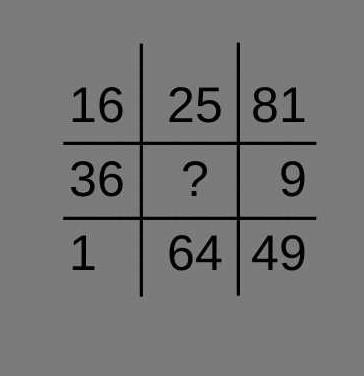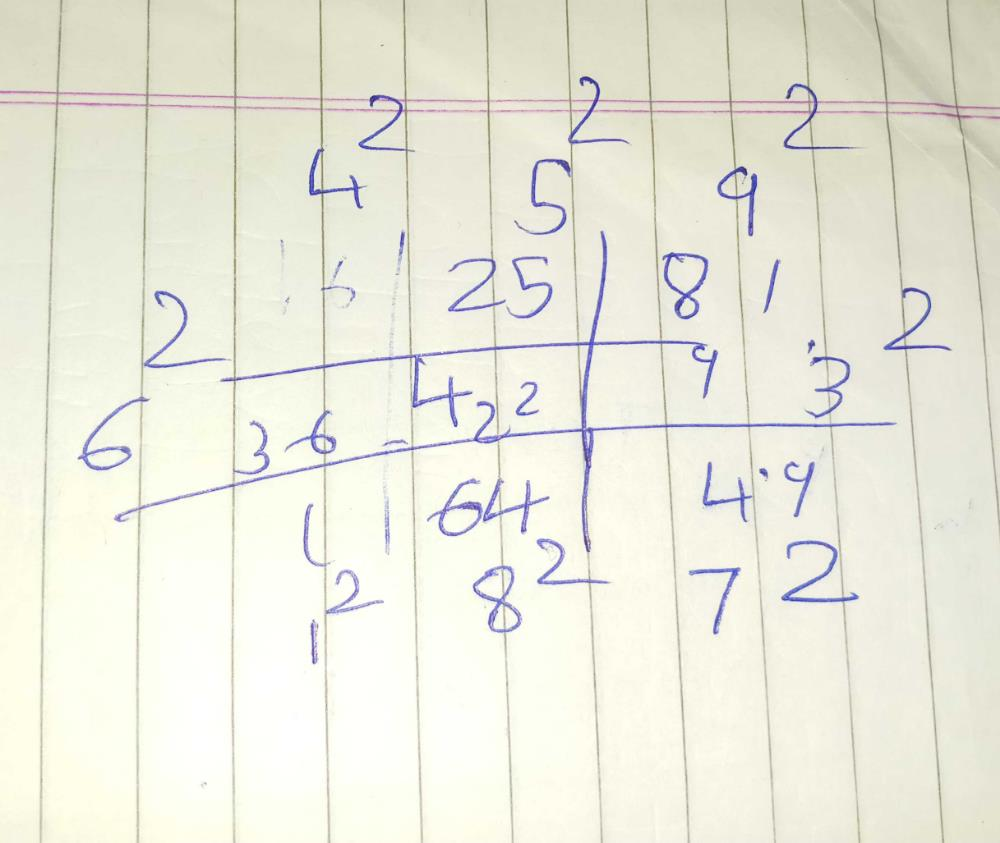Courses

# Find the missing number in the question given below?

## Class 10 Question

By Mysterio Man · Jun 19, 2020 ·Class 10
Mysterio Man answered Jun 19, 20204

Astha Shukla answered 3 weeks ago
4...

Mamta Devi answered Jun 21, 2020

Vaishnavi Saxena answered Jun 19, 2020
The answer is 4 as the squares of 1 to 9 is given except the no. 2 so the squqre of 2 will be 4 and answer is 4 .

Virendra Jha answered Jun 30, 2020
Root 16

Sarita Pandey answered Jun 19, 2020
I think that the correct answer is 4...
as there is square of every number from 1 to 9 in the block...
but the square of 2 i.e., 4 is Missing so 4 is the correct answer.

Drishti Kumari answered Jun 19, 2020
(2)^2 = 4 is the answer

Square 2 = 4

Harshit Agrawal answered 3 weeks ago

Poojashri M. K. answered Jun 30, 2020
Four.

Jitendra Saha answered Jun 30, 2020
There is square of every no. from 1 to 9 execept 2 so the middle blank will have 2² = 4

Anirudh M answered Jun 25, 2020
the given numbers are square numbers.only 2 is missing in the order so square of 2 is 4

Diana Nair answered Jun 21, 2020
I think the answer is 4 because all the numbers are squares of some numbers.But 2 square hadn't given so it should be 4.

Santosh Mishra answered Jun 29, 2020
4 is the answer for this question

Cyber Tamilzha answered 2 weeks ago

Guncha Jain answered 3 weeks ago

Jigisha Kiran answered 3 weeks agoMayank Raj answered Jul 02, 2020
100

Krishi Shah answered 2 weeks ago
36=4*9

Kohinoor Kale answered Jun 19, 2020
Answer is 4 i.e. 2 square

Armaan Gogoi answered 2 weeks ago
The answer must be 4 because squares of all the numbers n≥1 but n<10 are="" given="" except="" 2="" share="" that="" is="" 4.="" are="" given="" except="" 2="" share="" that="" is="">

Poorvi.m. Singh answered 6 days ago
4 is the answer as 2power2 must be filled there

Arushi Rohini Gautam answered 3 weeks ago
I think 4 is right answer

Aprajita Kumari answered 4 weeks ago
Square of all no except 2 is written so answer is 4

R C Kachhatia answered Jun 30, 2020
4 is the correct answer because all other number are square root till 9.

Divyanshu Pal answered 3 weeks ago
4 is the ans

Nikhil Kashyap answered 3 weeks ago
It'S 4 is missinG NumbeR

Poonam Fogat answered 3 days ago

Hey !!!
Hope this helps:)

Deepak Kumar answered 2 weeks ago
5

Tanisha Singh answered 3 days ago
Answer is 4 bcoz the squares of numbers are given here.. only the square of 2 is missing in this series...

Vinay Shinde answered 6 days ago
Yes bro .....4 is the right answer!!!

Amit Kumar answered 2 weeks ago
7

Uma Harinathan answered 2 weeks ago
Idk

81

Lovely Gods01 answered 6 days ago
Y

Rohan Giri answered 4 days ago
2

This discussion on Find the missing number in the question given below? is done on EduRev Study Group by Class 10 Students. The Questions and Answers of Find the missing number in the question given below? are solved by group of students and teacher of Class 10, which is also the largest student community of Class 10. If the answer is not available please wait for a while and a community member will probably answer this soon. You can study other questions, MCQs, videos and tests for Class 10 on EduRev and even discuss your questions like Find the missing number in the question given below? over here on EduRev! Apart from being the largest Class 10 community, EduRev has the largest solved Question bank for Class 10.